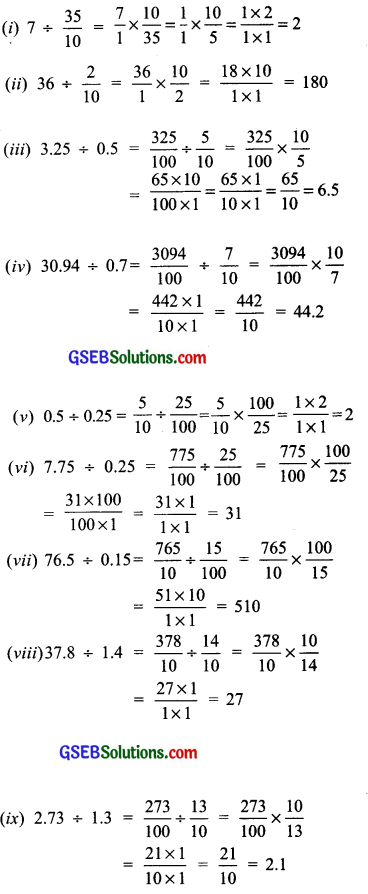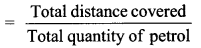# GSEB Solutions Class 7 Maths Chapter 2 Fractions and Decimals Ex 2.7

Gujarat Board GSEB Textbook Solutions Class 7 Maths Chapter 2 Fractions and Decimals Ex 2.7 Textbook Questions and Answers.

## Gujarat Board Textbook Solutions Class 7 Maths Chapter 2 Fractions and Decimals Ex 2.7Question 1.
Find:
(i) 0.4 ÷ 2
(ii) 0.35 ÷ 5
(iii) 2.48 ÷ 4
(iv) 65.4 ÷ 6
(v) 651.2 ÷ 4
(vi) 14.49 ÷ 7
(vii) 3.96 ÷ 4
(viii) 0.80 ÷ 5
Solution:
(i) 0.4 ÷ 2
Since $$\frac { 4 }{ 2 }$$ = 2 and there is one digit in the decimal part of the given decimal number.
∴ Place the decimal point in the quotient such that there is one digit to its right.
∴ 0.4 ÷ 2 = 0.2

(ii) 0.35 ÷ 5
Since 35 ÷ 5 = 7 and there are two digits in the decimal part of the given decimal number.
∴ Place the decimal point in the quotient such that there are two digits to its right.
∴ 0.35 ÷ 5 = 0.07

(iii) 2.48 ÷ 4
Since 248 ÷ 4 = 62 and there are two digits in the decimal part of the given decimal number.
∴ Place the decimal point in the quotient such that there are two digits to its right.
∴ 2.48 ÷ 4 = 0.62

(iv) 65.4 ÷ 6
Since 654 ÷ 6 = 109 and there is one digit in the decimal part of the given decimal number.
∴ Place the decimal point in the quotient such that there is one digit to its right.
∴ 65.4 ÷ 6 = 10.9

(v) 651.2 ÷ 4
Since 6512 ÷ 4 = 1628 and there is one digit in the decimal part of the given decimal number.
∴ Place the decimal point in the quotient such that there is one digit to its right.
∴ 651.2 ÷ 4 = 162.8

(vi) 14.49 ÷ 7
Since 1449 ÷ 7 = 207 and there are two digits in the decimal part of the given decimal number.
∴ Place the decimal point in the quotient such that there are two digits to its right.
∴ 14.49 ÷ 7 = 2.07

(vii) 3.96 ÷ 4
Since 396 ÷ 4 = 99 and there are two digits in the decimal part of the given decimal number.
∴ Place the decimal point in the quotient such that there are two digits to its right.
∴ 3.96 ÷ 4 = 0.99

(viii) 0.80 ÷ 5
Since 80 ÷ 5 = 16 and there are two digits in the decimal part of the given decimal number.
∴ Place the decimal point in the quotient such that there are two digits to its right.
∴ 0.80 ÷ 5 = 0.16Question 2.
Find:
(i) 4.8 ÷ 10
(ii) 52.5 ÷ 10
(iii) 0.7 ÷ 10
(iv) 33.1 ÷ 10
(v) 272.23 ÷ 10
(vi) 0.56 ÷ 10
(vii) 3.97 ÷ 10
Solution:
(i) 4.8 ÷ 10
As there is one zero in 10, the decimal point in the quotient is shifted to the left by one place.
∴ 4.8 ÷ 10 = 0.48

(ii) 52.5 ÷ 10
As there is one zero in 10, the decimal point in the quotient is shifted to the left by one place.
∴ 52.5 ÷ 10 = 5.25

(iii) 0.7 ÷ 10
As there is one zero in 10, the decimal point in the quotient is shifted to the left by one place.
∴ 0.7 + 10 = 0.07

(iv) 33.1 ÷ 10
As there is one zero in 10, the decimal point in the quotient is shifted to the left by one place.
∴ 33.1 ÷ 10 = 3.31

(v) 272.23 ÷ 10
As there is one zero in 10, the decimal point in the quotient is shifted to the left by one place.
∴ 272.23 ÷ 10 = 27.223

(vi) 0.56 ÷ 10
As there is one zero in 10, the decimal point in the quotient is shifted to the left by one place.
∴ 0.56 + 10 = 0.056

(vii) 3.97 ÷ 10
As there is one zero in 10, the decimal point in the quotient is shifted to the left by one place.
∴ 3.97 + 10 = 0.397Question 3.
Find:
(i) 2.7 ÷ 100
(ii) 0.3 ÷ 100
(iii) 0.78 ÷ 100
(iv) 432.6 ÷ 100
(v) 23.6 ÷ 100
(vi) 98.53 ÷ 100
Solution:
(i) 2.7 ÷ 100
∵ There are two zeros in 100, therefore, the decimal point in the quotient is shifted to the left by two places.
∴ 2.7 ÷ 100 = 0.027

(ii) 0.3 ÷ 100
∵ There are two zeros in 100, therefore, the decimal point in the quotient is shifted to the left by two places.
∴ 0.3 ÷ 100 = 0.003

(iii) 0.78 ÷ 100
∵ There are two zeros in 100, therefore, the decimal point in the quotient is shifted to the left by two places.
∴ 0.78 ÷ 100 = 0.0078

(iv) 432.6 ÷ 100
∵ There are two zeros in 100, therefore, the decimal point in the quotient is shifted to the left by two places.
∴ 432.6 ÷ 100 = 4.326

(v) 23.6 ÷ 100
∵ There are two zeros in 100, therefore, the decimal point in the quotient is shifted to the left by two places.
∴ 23.6 ÷ 100 = 0.236

(vi) 98.53 ÷ 100
∵ There are two zeros in 100, therefore, the decimal point in the quotient is shifted to the left by two places.
∴ 98.53 ÷ 100 = 0.9853

Question 4.
Find:
(i) 0 7.9 ÷ 1000
(ii) 26.3 ÷ 1000
(iii) 38.53 ÷ 1000
(iv) 128.9 ÷ 1000
(v) 0.5 4 ÷ 1000
Solution:
(i) 7.9 ÷ 1000
∵ There are three zeros in 1000, therefore, the decimal point in the quotient is shifted to the left by 3 places.
∴ 7.9 ÷ 1000 = 0.0079

(ii) 26.3 ÷ 1000
∵ There are three zeros in 1000, therefore, the decimal point in the quotient is shifted to the left by 3 places.
∴ 26.3 ÷ 1000 = 0.0263

(iii) 38.53 ÷ 1000
∵ There are three zeros in 1000, therefore, the decimal point in the quotient is shifted to the left by 3 places.
∴38.53 ÷ 1000 = 0.03853

(iv) 128.9 ÷ 1000
∵ There are three zeros in 1000, therefore, the decimal point in the quotient is shifted to the left by 3 places.
∴ 128.9 4 ÷ 1000 = 0.1289

(v) 0.5 ÷ 1000
∵ There are three zeros in 1000, therefore, the decimal point in the quotient is shifted to the left by 3 places.
∴ 0.5 4 ÷ 1000 = 0.0005Question 5.
Find:
(i) 7 ÷ 3.5
(ii) 36 ÷ 0.2
(iii) 3.25 ÷ 0.5
(iv) 30.94 ÷ 0.7
(v) 0.5 ÷ 0.25
(vi) 7.75 ÷ 0.25
(vii) 76.5 ÷ 0.15
(viii) 37.8 ÷ 1.4
(ix) 2.73 ÷ 1.3
Solution:Question 6.
A vehicle covers a distance of 43.2 km in 2.4 litres of petrol. How much distance will it cover in one litre of petrol?
Solution:
Total distance covered = 43.2 km
Quantity of petrol used = 2.4 litres
∴ Distance covered in one litre petrol= $$\frac { 43.2 }{ 2.4 }$$km
= [ $$\frac { 43.2 }{ 2.4 }$$ ÷ $$\frac { 24 }{ 10 }$$ ]km
= $$\frac { 432 }{ 10 }$$ x $$\frac { 10 }{ 24 }$$
= $$\frac { 18×1 }{ 1×1 }$$
= 18 km